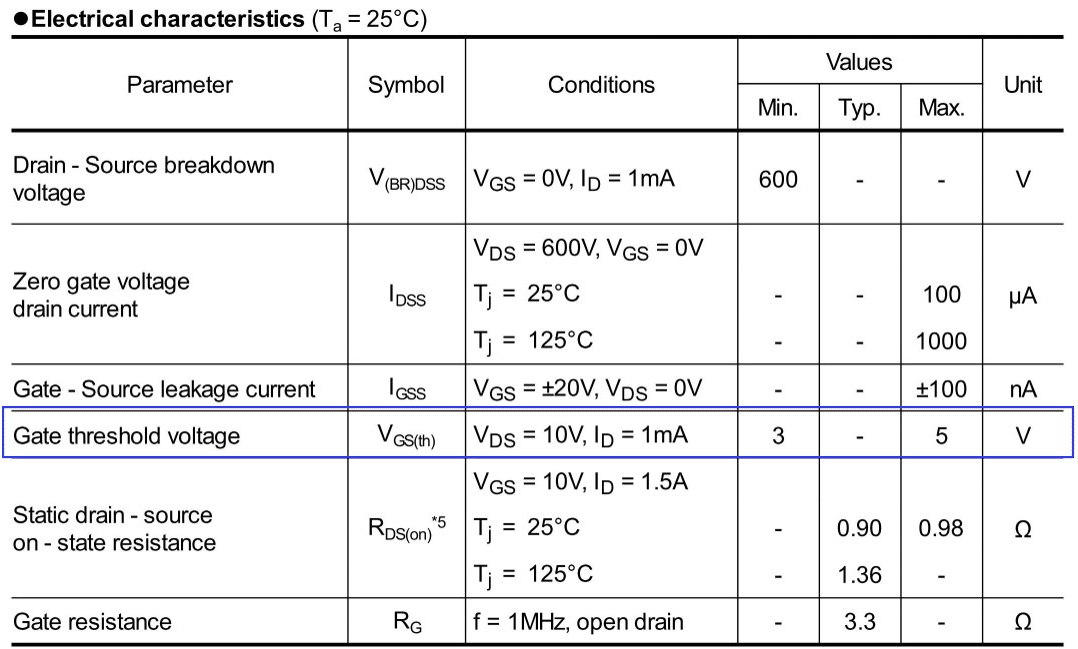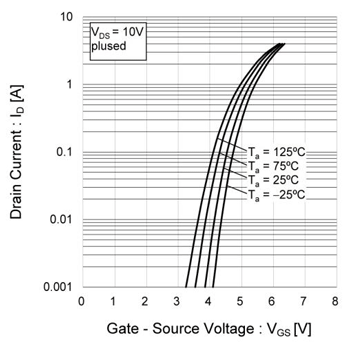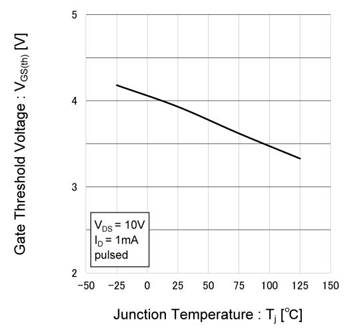# What are MOSFETs? – MOSFET Threshold Values, ID-VGS Characteristics, and Temperature Characteristics

2017.04.06

・The voltage at which a MOSFET turns on is called the gate threshold voltage.

・If VGS is constant, a rise in temperature will cause ID to increase, and so conditions of use must be considered carefully.

・Tj can be roughly estimated from a change in the value of VGS(th).

In succession to the preceding discussion of MOSFET switching characteristics, here we explain the gate threshold voltage, which is a crucial characteristic of MOSFETs, as well as the ID-VGS characteristics, and the temperature characteristics of each of these.

## MOSFET VGS(th)： Gate Threshold Voltage

The MOSFET VGS(th) or gate threshold voltage is the voltage between the gate and source that is needed to turn on the MOSFET. In other words, if VGS is at least as high as the threshold voltage, the MOSFET turns on.

Some persons may be wondering just how much of a current ID can be passed on this “MOSFET on” state. And it is true that ID changes depending on VGS. Speaking from the standpoint of the VGS(th) specification, if the conditions are not determined, a value for VGS(th) cannot be guaranteed; the datasheet for the MOSFET stipulates these conditions. This table is excerpted from the datasheet for the N-ch, 600 V, 4 A power MOSFET R6004KNX.The blue line surrounds information on VGS(th), and the conditions column indicates that conditions are VDS = 10 V and ID = 1 mA. Under these conditions, and at Ta = 25℃, VGS(th) is guaranteed to have minimum and maximum values of 3 V and 5 V.

In other words, as VGS is raised, the MOSFET begins to turn on (ID begins to flow), and when ID is 1 mA, the value of VGS is between 3 V and 5 V inclusive; this value is VGS(th). Various methods of expression are possible, but we can say that the MOSFET on state is defined as being when ID = 1 mA at VDS = 10 V, and the VGS at this time is taken to be VGS(th), the value of which is between 3 V and 5 V.

It should also be noted that voltages and currents that change according to some state, such as an input or output or a turning on or off of some function, are commonly called threshold values, and are not limited to MOSFETs.

## Temperature Characteristics of VGS(th) and ID-VGS

From the initial graph showing the ID-VGS characteristic, the VGS(th) for the MOSFET can be read off. The condition VDS = 10 V matches the stipulated condition. When ID is 1 mA, VGS is equal to VGS(th), and so the VGS when the curve for Ta = 25℃ intersects the 1 mA (0.001 A) line is approx. 3.8 V. The datasheet does not indicate a representative or typical value (indicated by “Typ”), and we see from the graph that the Typ value for VGS(th) is about 3.8 V. The graph value can in essence be regarded as the Typ value.

Next, we consider the ID-VGS characteristic. As the specification value for VGS(th), the value when ID = 1 mA is used, but in actual use, a 4 A MOSFET is unlikely to be used with ID at only 1 mA. For example, when an ID of 1 A at Ta = 25℃ is required, we see from the graph that the VGS is about 5.3 V.From the graph of the ID-VGS temperature characteristic, at high temperatures there is a tendency for ID to increase if VGS is constant. Taking as an example the previous condition that ID = 1 A at Ta = 25℃, at Ta = 75℃, an ID of about 1.5 A can be passed, and so the conditions of use must be considered carefully.

Returning to the gate threshold voltage, the temperature characteristic of VGS(th) is shown in a graph. As was seen from the ID-VGS graph, we see that at 25℃, VGS(th) is approx. 3.8 V. The temperature in this graph is Tj, but as indicated by the term “pulsed”, the data was obtained in pulsed tests, and it is permissible to assume that Tj ? Ta ? 25℃.

It is seen that the temperature characteristic is such that at high temperatures, VGS(th) tends to decline. This indicates that as the temperature rises, because VGS(th) declines, a larger ID can flow at a lower VGS. In other words, and as would be expected, this matches the ID-VGS temperature characteristic.

VGS(th) can be used to estimate Tj. The VGS(th) temperature characteristic is linear, and so a proportionality coefficient can be calculated, and the increase in temperature can be calculated from the amount of change in VGS(th).